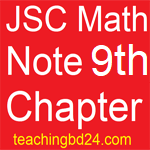JSC Math Note2 9th Chapter Pythagoras theorem

JSC Math Note2 9th Chapter Pythagoras theorem. Sixth-century Greek philosopher Pythagoras identified a necessary characteristic of the right triangle. This feature of the right triangle is known as Pythagoras. It is said that before the birth of Pythagoras, the use of this feature of the right triangle was used in the Egyptian and Babylonian era. In this chapter, we will discuss this feature of the right triangle. The arms of the right triangle are known as specials. The opposite arm of the right angle and the adjacent adjoining land, respectively, land and improvement. The current chapter will discuss the relationship between these three arm lengths.

JSC Math Note2 9th Chapter Pythagoras theoremThe quadrilateral is divided into two triangle lines with a quadrilateral. Therefore, the area of the quadrilateral is equal to the sum of the area of the triangle. In the previous class, we learned to calculate the area of the square and rectangle. Again, the land and height of the rectangles and parallels are the same, but the areas of the given fields are equal. Below you will discuss the area of the rhombus and the topic area.

Students at the end of the section:

The Pythagorean theorem can verify and prove. If the three sides of the triangle are given length, then the triangle is able to verify whether it is the right angle.

Using Pythagoras formula can solve the problem

The gathering of the well-defined object of real or thought is called set. Examples of the well-defined set of the first five letters of the English alphabet, countries of Asia continent, natural numbers, etc. No member is under consideration. There is no specific set which is not included in the set. Repeat the members of the set and No order The set element for each member of the set. (Businessman) It is said. The set is usually the uppercase of the English alphabet.

teachingbd24.com is such a website where you would get all kinds of necessary information regarding educational notes, suggestions and questions’ patterns of school, college, and madrasahs. Particularly you will get here special notes of physics that will be immensely useful to both students and teachers. The builder of the website is Mr. Md. Shah Jamal Who has been serving for 33 years as an Asst. Professor of BAF Shaheen College Dhaka. He expects that this website will meet up all the needs of Bengali version learners /students. He has requested both concerned students and teachers to spread this website home and abroad.